# Methods and formulas for event predictions in Fit Poisson Model

Select the method or formula of your choice.

## Fitted and predicted values

Different models have different link functions. To calculate the prediction, invert the link function for the model. The inverse functions are in this table.

Model Link Function Formula for Prediction
Binomial Logit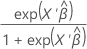Binomial Normit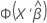Binomial Gompit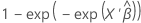Poisson Natural log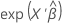Poisson Square root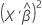Poisson Identity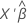### Notation

TermDescription
exp(·) the exponential function
Φ(·) the cumulative distribution function of the normal distribution
X' the transpose of the vector of points to predict for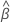the vector of estimated coefficients

## Standard error of fitted values and predictions

In general, the standard error of the fit has the following form:
The following formulas give the standard error of the fit for different link functions:
Logit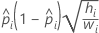Normit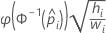Gompit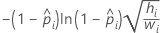Note the following relationship that applies to the formulas in the table:

where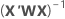is from the training data only when there is a test data set for validation.

### Notation

TermDescription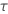1, for the binomial and Poisson models
xithe vector of a design point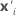the transpose of xi
Xthe design matrix
Wthe weight matrix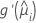the first derivative of the link function evaluated atthe predicted mean response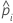the predicted probability for the design point in a binary logistic model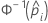the inverse cumulative distribution function of the standard normal distribution for the predicted probability in a binary logistic model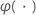the probability density function of the standard normal distribution

## Confidence limits for fits and predictions

The confidence limits use the Wald approximation method. The following is the general formula for a 100(1 − α)% two-sided confidence interval:

The following table gives specific formulas for the different model types and link functions:
Type Link Standard error of the fit
Binary logistic Logit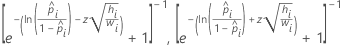Binary logistic Normit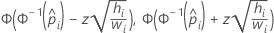Binary logistic Gompit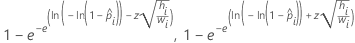Poisson Log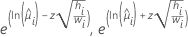Poisson Square root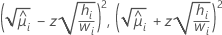Poisson Identity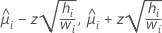Note the following relationship that applies to the formulas in the table:

whereis from the training data only when there is a test data set for validation.

### Notation

TermDescription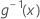the inverse of the link function evaluated at x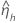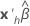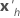the transpose of the vector of the predictorsthe vector of estimated coefficients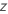the value of the inverse cumulative distribution function for the normal distribution evaluated at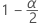αthe significance level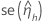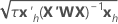Xthe design matrix
Wthe weight matrix1, for binomial and Poisson modelsthe predicted probability for the design point in a binary logistic modelthe inverse cumulative distribution function of the standard normal distribution for the predicted probability in a binary logistic model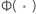the cumulative distribution function of the standard normal distribution
By using this site you agree to the use of cookies for analytics and personalized content.  Read our policy